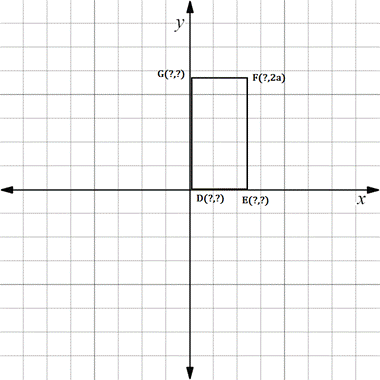Chapter 10.CR, Problem 28CRElementary Geometry For College St...

7th Edition
Alexander + 2 others
ISBN: 9781337614085

Solutions

Chapter
SectionElementary Geometry For College St...

7th Edition
Alexander + 2 others
ISBN: 9781337614085
Textbook Problem

In Review Exercise 27 to 30, supply the missing coordinates for the vertices, using as few variables as possible.Rectangle D E F G with D G = 2 · D E

To determine

To find:

The missing coordinates for the vertices.

Explanation

The given figure is shown below.

From the figure, the following observations are made.

Since, the vertex D is at the origin.

The coordinates of D0, 0.

Since, the vertical distance from D to E is 2a as its y-coordinate is 2a.

Also the distance DG=2a.

In the problem, it is given that DG=2·DE

2a=2·DE

Cancelling 2 on both sides,

DE=a

Since DE is the horizontal distance, the x-coordinate of E is a.

In the E vertex, observe where it lies on y- axis.

Since, it is at 0, the vertex E has the coordinates a, 0

Still sussing out bartleby?

Check out a sample textbook solution.

See a sample solution

The Solution to Your Study Problems

Bartleby provides explanations to thousands of textbook problems written by our experts, many with advanced degrees!

Get Started

In Problems 7-20, find each limit, if it exists.

Mathematical Applications for the Management, Life, and Social Sciences

Absolute Value Evaluate each expression. 69. (a) ||6||4|| (b) 1|1|

Precalculus: Mathematics for Calculus (Standalone Book)

Which number is the best choice for the value of a in the following graph? a) e b) 1 c) 2 d) 3

Study Guide for Stewart's Single Variable Calculus: Early Transcendentals, 8th